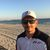Qlik NPrinting Discussions

Discussion Board for collaboration on Qlik NPrinting.

Announcements
Join us for live office hours! Q&A with Qlik on Dec 14 at 10am EST: Refining Reports and Visualizations
cancel
Showing results for
Did you mean:Contributor II

Pixel Perfect - Calculated Total

Hi all,

I have a table in Pixel Perfect that contains Sales, Budget, Variance to Budget in \$, and Variance to Budget in % as the four columns. The problem arises in the totals.

I can sum the first three columns with no problems using the Summary tool, but Summing (or averaging) a column of percentages does not work properly - I need to be able to calculate the Total Variance to Budget in % as a product of the Total Variance to Budget in \$ divided by the Total Budget. Is there a way to do this?

If this is impossible I can create a new table within Sense the contains only the totals, and insert that below, however it would be much better if I could do the above as I have to do this for multiple tables.

Many thanks,

Connor

Labels (2)

• Qlik Sense

1 Solution

Accepted SolutionsEmployee

Hi,

You cannot calculate the total of a percentage as sum of the single percentages of the column. You have to create a new calculated field in the PixelPerfect template:

1. right click on the table row in the levels node and select Add a calculated field
2. in the expression insert the formula sum(field1)/sum(field2) (adapt the formula based on your needs)
3. drag and drop the new calculated field in the template

In the following example I calculate the percentage of each row compared with the total sum of all rows:Best Regards,

Ruggero

Best Regards,
Ruggero
---------------------------------------------
When applicable please mark the appropriate replies as CORRECT. This will help community members and Qlik Employees know which discussions have already been addressed and have a possible known solution. Please mark threads with a LIKE if the provided solution is helpful to the problem, but does not necessarily solve the indicated problem. You can mark multiple threads with LIKEs if you feel additional info is useful to others.
4 RepliesMVP

Since you are able to calculate total for Sales and Budget why dont you use those to calculate % on total?

cheers Lech, When applicable please mark the correct/appropriate replies as "solution" (you can mark up to 3 "solutions". Please LIKE threads if the provided solution is helpful to the problem.Contributor II
Author

Hi Lech,

That's actually what I'm asking how to do/if it is possible. Apologies if the question was a simple one, I am new to Pixel Perfect.

How would I do this?Employee

Hi,

You cannot calculate the total of a percentage as sum of the single percentages of the column. You have to create a new calculated field in the PixelPerfect template:

1. right click on the table row in the levels node and select Add a calculated field
2. in the expression insert the formula sum(field1)/sum(field2) (adapt the formula based on your needs)
3. drag and drop the new calculated field in the template

In the following example I calculate the percentage of each row compared with the total sum of all rows:Best Regards,

Ruggero

Best Regards,
Ruggero
---------------------------------------------
When applicable please mark the appropriate replies as CORRECT. This will help community members and Qlik Employees know which discussions have already been addressed and have a possible known solution. Please mark threads with a LIKE if the provided solution is helpful to the problem, but does not necessarily solve the indicated problem. You can mark multiple threads with LIKEs if you feel additional info is useful to others.Contributor II
Author

Hi Ruggero,

Thanks, that worked perfectly! Appreciate it.Community Browser